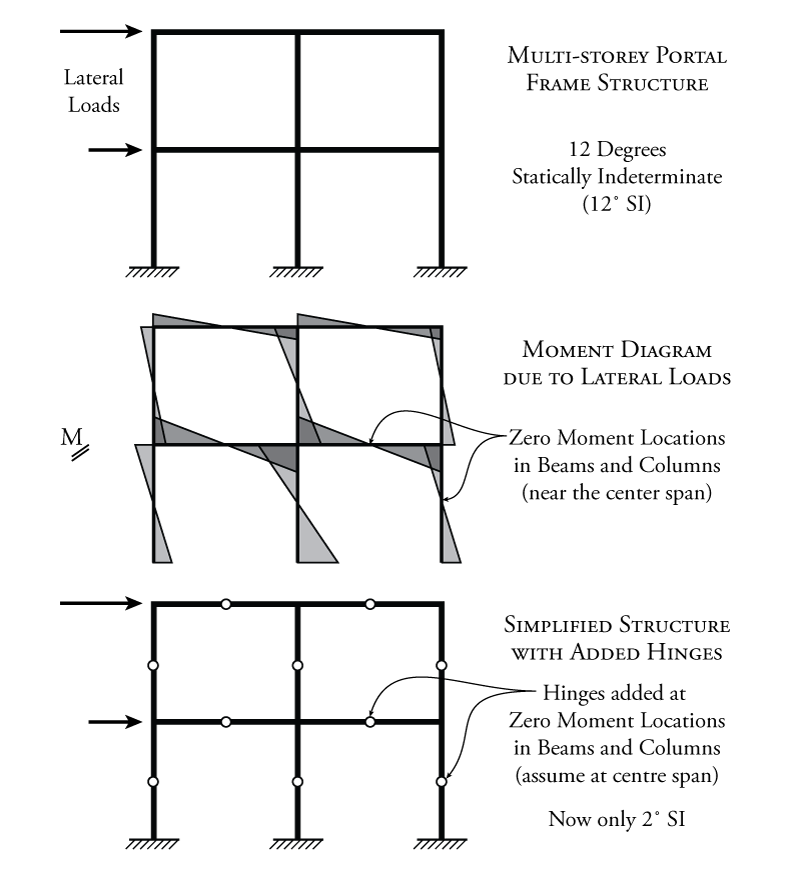# 7.1 Introduction

Now that we have a thorough background in the analysis of determinate structures, the rest of this book will focus on the analysis of indeterminate structures. Recall that indeterminate structures are those that we cannot analyse using the three equilibrium equations alone.

One of the most common analysis cases in the design of building structures is the analysis of a multi-storey portal frame subject to lateral loads. An example of such a frame is shown at the top of Figure 7.1. These types of lateral loadings may be caused by earthquake or wind forces. The frame shown in the figure has two storeys and two bays (the number of single portal frames in the horizontal direction). If we want to analyse such a structure to determine the moments and shears for design, we will immediately realize that this structure is highly indeterminate. We can calculate that the frame is $\ang{12}$ indeterminate using the methods from Chapter 2.

We would like to have some way to quickly analyse this type of structure. But, to do so, we will have to make some good assumptions about it's behaviour. This means that the resulting shears and moments will not be exact, but will have some built-in error due to our assumptions. An alternate method to analyse such a structure more precisely will be covered later on in Chapter 10#####.Figure 7.1: Adding Hinges to Approximate the Behaviour of an Indeterminate Portal Frame - Fixed Base